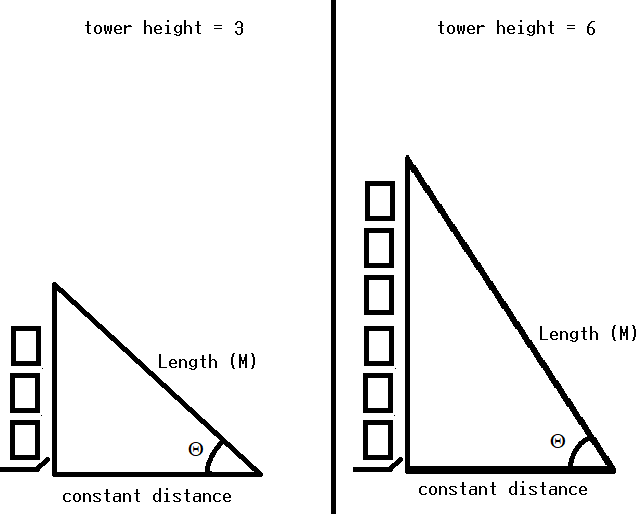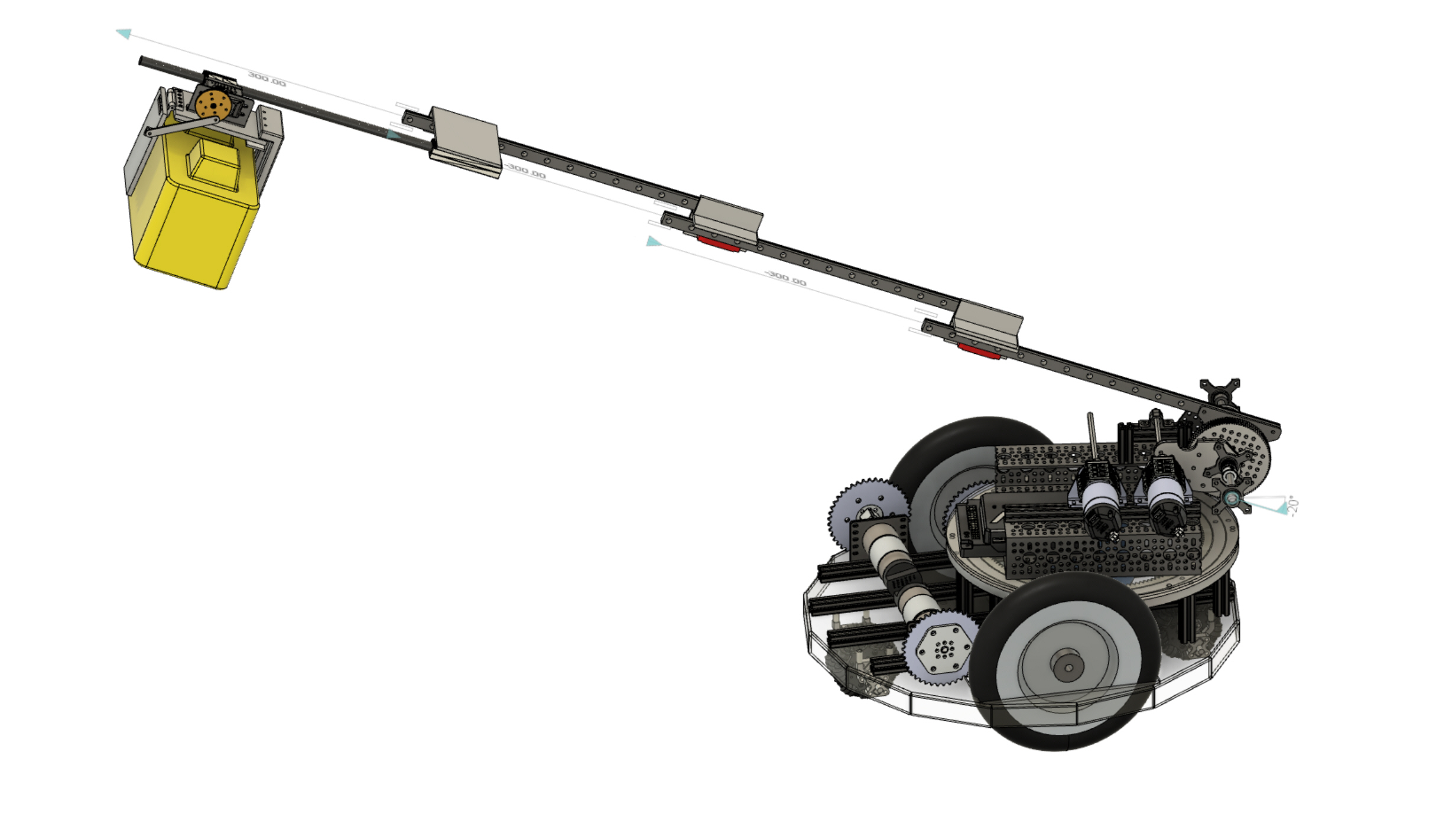# Extend to Tower Height and Retract from Tower

Tags: think and control
Personhours: 5
Extend to Tower Height and Retract from Tower By Cooper

### Task: Develop the controller so that it can extend to tower height

Since we have decided to move onto using 2 controllers, we can have more room for optimizations and shortcuts/ articulations. One such articulation is the extendToTowerHeight articulation . It takes a value for the current tower height and when a button is pushed, it extends to just over that height, so a block can be placed. This happened in 2 different segments of development.

The first leg of development was the controller portion. Since this was the first time we had used a 2nd controller, we ran into an unexpected issue. We use a method called toggleAllowed() that Tycho wrote many years ago for our non-continuous inputs. It worked just fine until we passed it the second controllers inputs, as then it would not register any input. The problem was in the method, as it worked on an array of the buttons on the controller to save states, and there was conflict with the first controller. So, we created a new array of indexes for the second controller, and made it so in the method call you pass it the gpID (gamepad ID), which tells it which of those index arrays to use. Once that was solved, we were able to successfully put incrementTowerHeight() on the y button and decrementTowerHeight() on the x button. The current tower height is then spit out in telemetry for the second driver to see.

Then came the hard part of using that information. After a long discussion, we decided to with a extendToTowerHeight() that has a constant distance, as having a sensor for distance to the build platform would have too many variables in what direction i t should be in, and having it be constant means the math works out nicely. So this is how it would look:Now, we can go over how we would find all of these values. To start, we can look at the constant distance measure, and to be perfectly honest it is a completely arbitrary value. We just placed the robot a distance that looked correct away from the center of the field. This isn’t that bad, as A) we can change it, and B) it doesn’t need to be calculated. The driver just needs to practice with this value and that makes it correct. In the end we decided to go with ~.77 meters.

Then before we moved on we decided to calculate the TPM (Ticks Per Meter) of the extension of the arm, and the TPD (Ticks Per Degree) of the elbow, as it is necessary for the next calculations. For the TPM, we busted out a ruler and measure the extension of different positions in both inches (which were converted into Meters) and the tick value, then added them all up respectively and made a tick per meter ratio. In the end, we ended up with a TPM of 806/.2921. We did similar with the TPD, just with a level, and got 19.4705882353. With a quick setExtendABobLengthMeters() and a setElbowTargetAngle() method, it was time to set up the math. As can be seen in the diagram, we can think of the entire system as a right triangle. We know the opposite side (to theta) length, as we can multiply the tower height by the height, and we know the adjacent side’s length, as it is constant. Therefore, we can use the Pythagorean theorem to calculate the distance, in meters, of the hypotenuse.

``` hypotenuse = Math.sqrt(.76790169 + Math.pow(((currentTowerHeight+1)* blockHeightMeter),2));//in meters ```

``` From that, we can calculate the theta using a arccosine function of the adjacent / hypotenuse. In code, it ended up looking like this: .setElbowTargetAngle(Math.toDegrees(Math.acos(0.8763/ hypotenuse))); ```

Then we set the extension to be the hypotenuse:

``` setExtendABobLengthMeters(hypotenuse-.3683); ```

While it has yet to be seen its effectiveness, it should at the very least function well, as shown in our tests. This will help the drivers get into the general area of the tower, so they can worry more about the fine adjustments. For a more visual representation, here is the position in CAD:### Next Steps:

We need to work on 2 main things. Tuning is one, as while it is close, it’s not perfect. The second thing to work on is using a custom vision program to automatically detect the height of the tower. This would take all the stress off the drivers.

Date | December 28, 2019